Courses

# Irodov Solutions: The First Law of Thermodynamics Heat Capacity- 1 Notes | EduRev

## JEE : Irodov Solutions: The First Law of Thermodynamics Heat Capacity- 1 Notes | EduRev

The document Irodov Solutions: The First Law of Thermodynamics Heat Capacity- 1 Notes | EduRev is a part of the JEE Course Physics For JEE.
All you need of JEE at this link: JEE

Q. 26. Demonstrate that the interval energy U of the air in a room is independent of temperature provided the outside pressure p is constant. Calculate U, if p is equal to the normal atmospheric pressure and the room's volume is equal to V = 40 m3

Solution. 26. Internal energy of air, treating as an ideal gas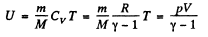(1)

Using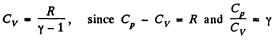Thus at constant pressure U = constant, because the volume of the room is a constant.

Puting the value of p = Patm and V in Eq. (1), we get U = 10 MJ.

Q. 27. A thermally insulated vessel containing a gas whose molar mass is equal to M and the ratio of specific heats Cp/Cv  = γ  moves with a velocity v. Find the gas temperature increment resulting from the sudden stoppage of the vessel.

Solution. 27. From eneigy conservation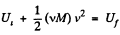or,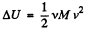(1)

But from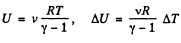(trom the previous problem)     (2)

Hence from Eqs. (1) and (2).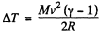Q. 28. Two thermally insulated vessels 1 and 2 are filled with air and connected by a short tube equipped with a valve. The volumes of the vessels, the pressures and temperatures of air in them are known (V1, p1, T1 and V2, p2, T2). Find the air temperature and pressure established after the opening of the valve.

Solution. 28. On opening the valve, the air will flow from the vessel at heigher pressure to the vessel at lower pressure till both vessels have the same air pressure. If this air pressure is p, the total volume of the air in the two vessels will be (V1 + V2). Also if v1 and v2 be the number of moles of air initially in the two vessels, we have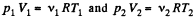(1)

After the air is mixed up, the total number of moles are (v1 + v2) and the mixture is at temperature T.

Hence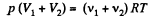(2)

Let us look at the two portions of air as one single system. Since this system is contained in a thermally insulated vessel, no heat exchange is involved in the process. That is, total heat transfer for the combined system Q = 0

Moreover, this combined system does not perform mechanical work either. The walls of the containers are rigid and there are no pistons etc to be pushed, looking at the total system, we know A = 0.

Hence, internal energy of the combined system docs not change in the process. Initially energy of the combined system is equal to the sum of internal energies of the two portions of air :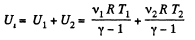(3)

Final internal energy of (n1 + n2) moles of air at temperature T is given by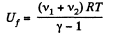(4)

Therefore, Ui = Uf implies :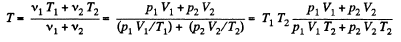From (2), therefore, final pressure is given by :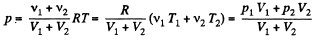This process in an example of free adiabatic expansion of ideal gas.

Q. 29. Gaseous hydrogen contained initially under standard conditions in a sealed vessel of volume V = 5.0 l was cooled by ΔT = 55 K . Find how much the internal energy of the gas will change and what amount of heat will be lost by the gas.

Solution. 29. By the first law of thermodynamics,

Q = ΔU + A

Here A = 0, as the volume remains constant,

So,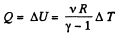From gas law,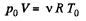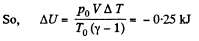Hence amount of heat lost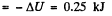Q. 30. What amount of heat is to be transferred to nitrogen in the isobaric heating process for that gas to perform the work A = 2.0 J?

Solution. 30. By the first law of thermodynamics  Q = ΔU + A

But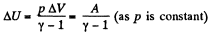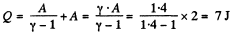Q. 31. As a result of the isobaric heating by ΔT = 72 K one mole of a certain ideal gas obtains an amount of heat Q = 1.60 kJ. Find the work performed by the gas, the increment of its internal energy, and the value of γ = Cp/Cv.

Solution. 31. Under isobaric process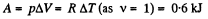From the first law of thermodynamics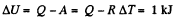Again increment in internal energy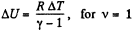Thus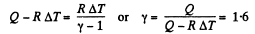Q. 32. Two moles of a certain ideal gas at a temperature T0 = 300 K were cooled isochorically so that the gas pressure reduced n = 2.0 times. Then, as a result of the isobaric process, the gas expanded till its temperature got back to the initial value. Find the total amount of heat absorbed by the gas in this process.

Solution. 32. Let v = 2 moles of the gas. In the first phase, under isochoric process, A1 = 0, therefore from gas law if pressure is reduced n times so that temperature i.e. new temperature becomes T0/n.

Now from first law of thermodynamics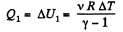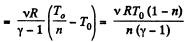During the second phase (under isobaric process),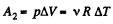Thus from first law of thermodynamics :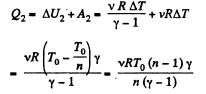Hence the total amount of heat absorbed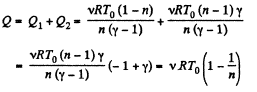Q. 33. Calculate the value of γ = Cp/Cv  for a gaseous mixture consisting of v1  = 2.0 moles of oxygen and v2 = 3.0 moles of carbon dioxide. The gases are assumed to be ideal.

Solution. 33. Total no. of moles of the mixture v = v1 + v2

At a certain temperature,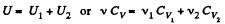Thus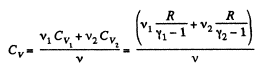Similarly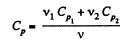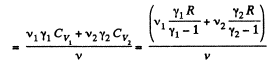Thus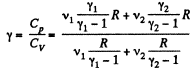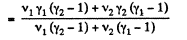Q. 34. Find the specific heat capacities cv and cp for a gaseous mixture consisting of 7.0 g of nitrogen and 20 g of argon. The gases are assumed to be ideal.

Solution. 34. Ttoto. previous problem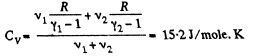and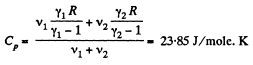Now molar mass of the mixture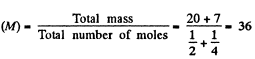Hence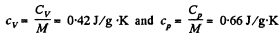Q. 35. One mole of a certain ideal gas is contained under a weightless piston of a vertical cylinder at a temperature T. The space over the piston opens into the atmosphere. What work has to be performed in order to increase isothermally the gas volume under the piston it times by slowly raising the piston? The friction of the piston against the cylinder walls is negligibly small.

Solution. 35. Let 5 be the area of the piston and F be the force exerted by the external agent Then, F + p S - p0S (Fig.) at an arbitrary instant of time. Here p is the pressure at the instant the volume is V. (Initially the pressure inside is p0)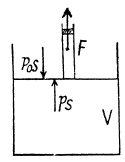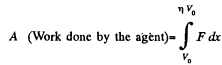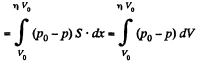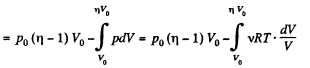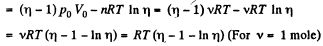Q. 36. A piston can freely move inside a horizontal cylinder closed from both ends. Initially, the piston separates the inside space of the cylinder into two equal parts each of volume V0, in which an ideal gas is contained under the same pressure P0  and at the same temperature. What work has to be performed in order to increase isothermally the volume of one part of gas η times compared to that of the other by slowly moving the piston?

Solution. 36. Let the agent move the piston to the right by x. In equilibirium position,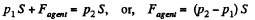Work done by the agent in an infinitesmal change dx is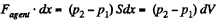By applying pV = constant, for the two parts,So,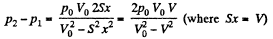When the volume of the left end is η times the volume of the right end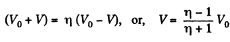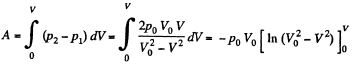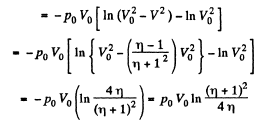Q. 37. Three moles of an ideal gas being initially at a temperature T0 = 273 K were isothermally expanded n = 5.0 times its initial volume and then isochorically heated so that the pressure in the final state became equal to that in the initial state. The total amount of heat transferred to the gas during the process equals Q = 80 kJ. Find the ratio γ = Cp/Cv for this gas.

Solution. 37. In the isothermal process, heat transfer to the gas is given by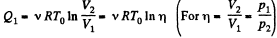In the isochoric process, A = 0

Thus heat transfer to the gas is given by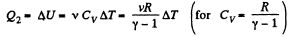But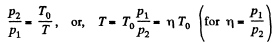or,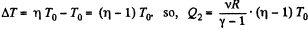Thus, net heat transfer to the gas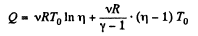or,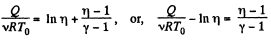or,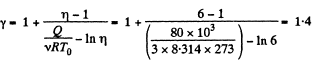Q. 38. Draw the approximate plots of isochoric, isobaric, isothermal, and adiabatic processes for the case of an ideal gas, using the following variables:

(a) p, T; (b) V, T.

Solution. 38. (a) From ideal gas law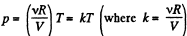For isochoric process, obviously k = constant, thus p = kT, represents a straight line passing through the origin and its slope becomes k.
For isobaric process p = constant, thus on p - T curve, it is a horizontal straight line parallel to T - axis, if T is along horizontal (or x - axis)

For isothermal process, T = constant, thus on p - T curve, it represents a vertical straight line if T is taken along horizontal (or x - axis)

For adiabatic process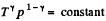After diffrentiating, we get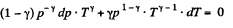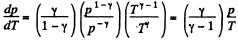The approximate plots of isochoric, isobaric, isothermal, and adiabatic processess are drawn in the answersheet.

(b) As p is not considered as variable, we have from ideal gas law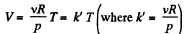On V - T co-ordinate system let us, take T along x - axis.
For isochoric process V = constant, thus k' = constant and V = k'T obviously represents a straight line pasing through the origin of the co-ordinate system and k' is its slope.
For isothermal process T = constant. Thus on the stated co- ordinate system it represents a straight line parallel to the V - axis.
For adiabatic process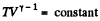After differentiating, we get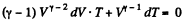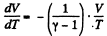The approximate plots of isochoric, isobaric, isothermal and adiabatic processess are drawn in the answer sheet.

Q. 39. One mole of oxygen being initially at a temperature T0 = 290 K is adiabatically compressed to increase its pressure η = 10.0 times. Find:
(a) the gas temperature after the compression;
(b) the work that has been performed on the gas.

Solution. 39. According to T - p relation in adiabatic process,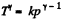(where k = constant)

and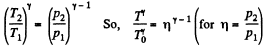Hence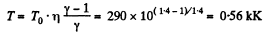(b) Using the solution of part (a), sought work done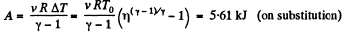Q. 40. A certain mass of nitrogen was compressed η = 5.0 times (in terms of volume), first adiabatically, and then isothermally. In both cases the initial state of the gas was the same. Find the ratio of the respective works expended in each compression.

Solution. 40. Let (p0, V0,T0) be the initial state of the gas.

We know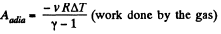But from the equation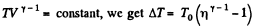Thus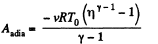On the other hand, we know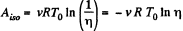(work done by the gas)

Thus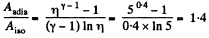Q. 41. A heat-conducting piston can freely move inside a closed thermally insulated cylinder with an ideal gas. In equilibrium the piston divides the cylinder into two equal parts, the gas temperature being equal to T0 . The piston is slowly displaced. Find the gas temperature as a function of the ratio η of the volumes of the greater and smaller sections. The adiabatic exponent of the gas is equal to γ.

Solution. 41. Since here the piston is conducting and it is moved slowly the temperature on the two sides increases and maintained at the same value.
Elementary work done by the agent = Work done in compression - Work done in expansion

i.e. dA - p2 dV - p1 dV = (p2 - P1) dV

where p1 and p2 are pressures at any instant of the gas on expansion and compression side respectively.

From the gas law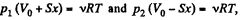for each section

(x is the displacement of the piston towards section 2)

So,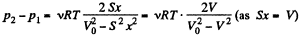So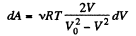Also, from the first law of thermodynamics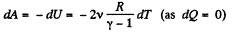So, work done on the gas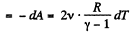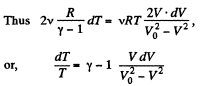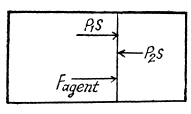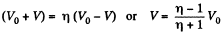On integrating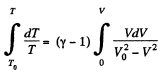or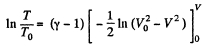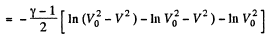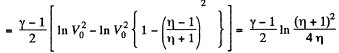Hence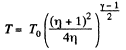Q. 42. Find the rate v with which helium flows out of a thermally insulated vessel into vacuum through a small hole. The flow rate of the gas inside the vessel is assumed to be negligible under these conditions. The temperature of helium in the vessel is T = 1,000 K.

Solution. 42. From energy conservation as in the derivation of Bernoulli theorem it reads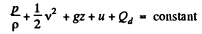(1)

In the Eq. (1) u is the internal eneigy per unit mass and in this case is the thermal eneigy per unit mass of the gas. As the gas vessel is thermally insulated Qd = 0, also in our case.

Just inside the vessel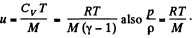Inside the vessel v = 0 also. Just outside p = 0, and u = 0. Ingeneral gz is not very significant for gases.

Thus applying Eq. (1) just inside and outside the hole, we get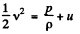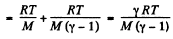Hence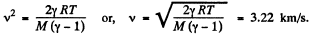Note : The velocity here is the velocity of hydrodynamic flow of the gas into vaccum. This requires that the diameter of the hole is not too small (D > mean free path l). In the opposite case (D < < l) the flow is called effusion. Then the above result does not apply and kinetic theory methods are needed.

Q. 43. The volume of one mole of an ideal gas with the adiabatic exponent γ is varied according to the law V = a/T, where a is a constant. Find the amount of heat obtained by the gas in this process if the gas temperature increased by ΔT.

Solution. 43. The differential work done by the gas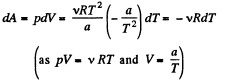So,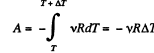From the Grst law of thermodynamics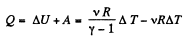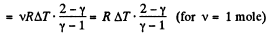Offer running on EduRev: Apply code STAYHOME200 to get INR 200 off on our premium plan EduRev Infinity!

## Physics For JEE

187 videos|516 docs|263 tests

,

,

,

,

,

,

,

,

,

,

,

,

,

,

,

,

,

,

,

,

,

;